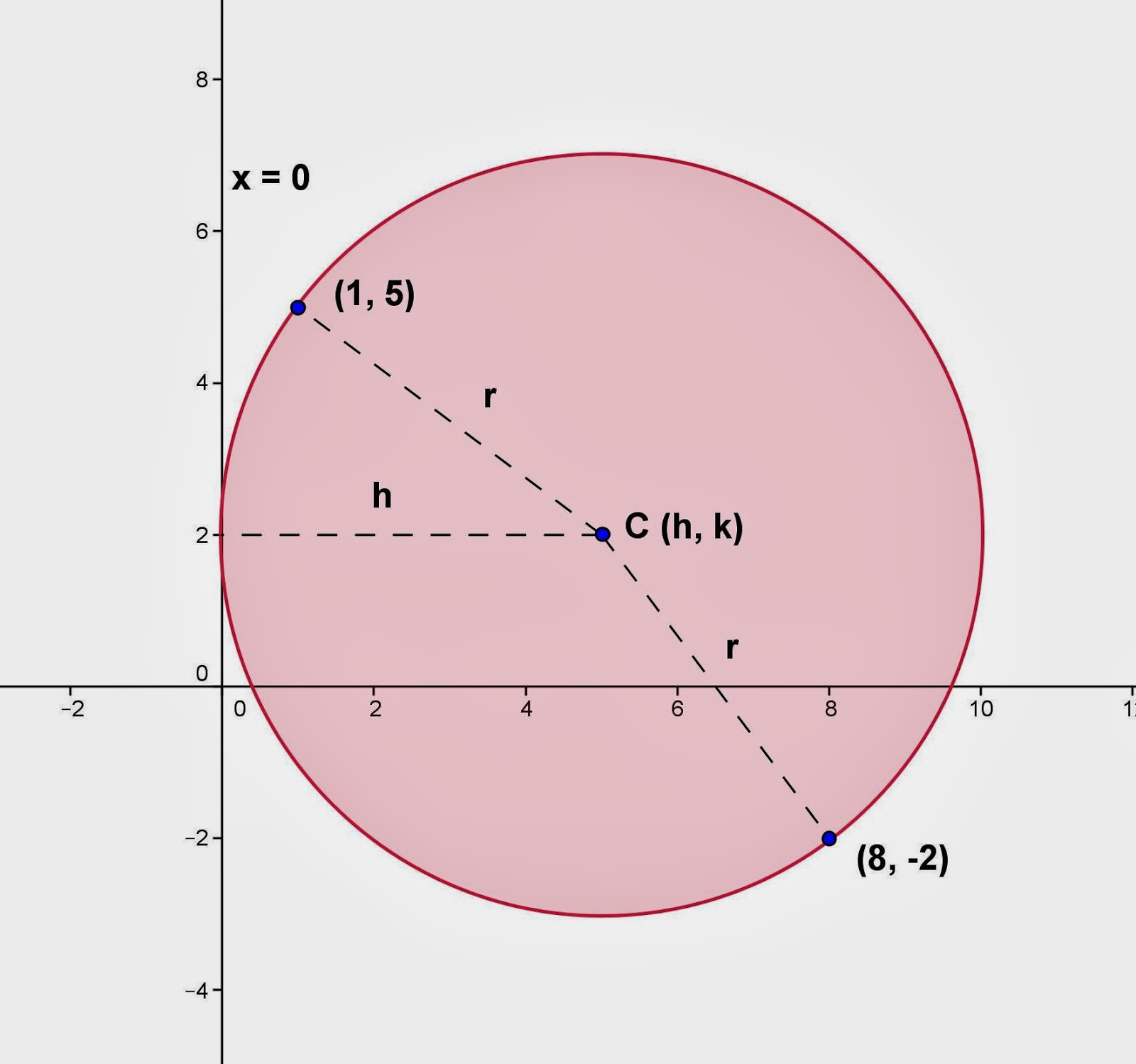## Thursday, January 30, 2014

### Finding Equation - Circle, 8

Category: Analytic Geometry, Plane Geometry, Algebra

"Published in Newark, California, USA"

Find the equation of a circle which is tangent to the y-axis and passes through the points (1, 5) and (8, -2).

Solution:

To illustrate the problem, it is better to draw the figure as followsA circle is tangent to the y-axis and passes thru (1, 5) and (8, -2). (Photo by Math Principles in Everyday Life)

Since the given circle is tangent to the y-axis, then the radius is equal to h which is the x-coordinate of the center of a circle.

Since the two points of a circle are given, then we can use the distance of two points formula in order to get the radius of a circle as follows

We need to get another equation because the above equation consists of two variables as follows

Substitute the value of h from equation (1) to equation (2), we have

After equating each factor to zero, the values of k are 10 and 2.

If k = 10, then

Hence, the center of a circle is C (13, 10) and its radius is r = h = 13.

Therefore, the equation of a circle is

If k = 2, then

Hence, the center of a circle is C (5, 2) and its radius is r = h = 5.

Therefore, the equation of a circle is